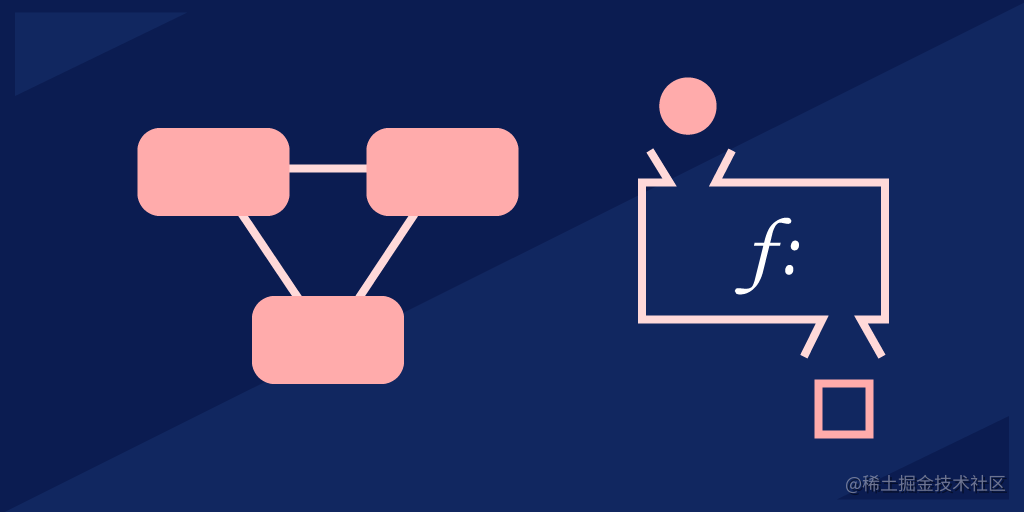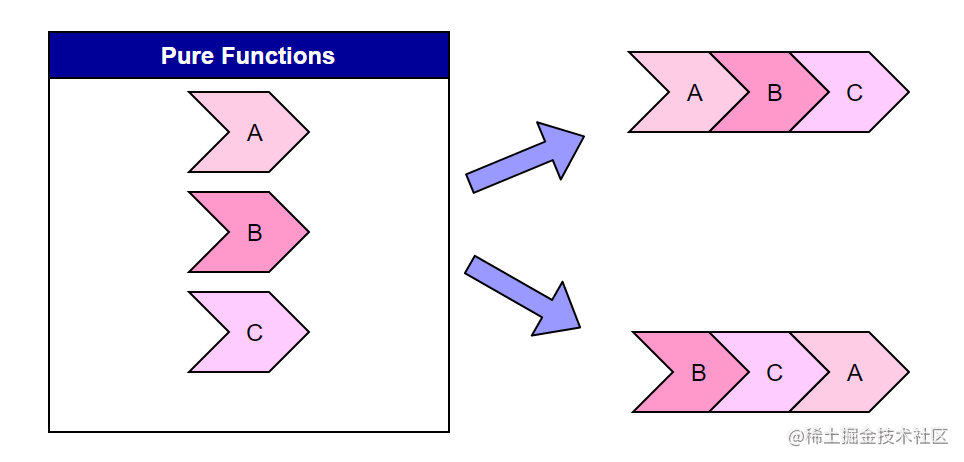# [译] 函数式编程 —— 使用 Python、JavaScript 和 Java 描述# 函数式编程 —— 使用 Python、JavaScript 和 Java 描述• 函数式编程是什么
• 函数式编程语言
• 函数式编程的相关概念
• 函数式编程 —— 使用 Python
• 函数式编程 —— 使用 JavaScript
• 函数式编程 —— 使用 Java
• 接下来学什么

## 函数式编程是什么## 函数式编程的优点

• 调试简单：纯函数和不可变的数据使查找设置变量值的位置变得容易。影响纯函数的因素更少，因此能让你更容易发现有 Bug 的部分。
• 延迟计算：函数式程序只在计算结果被需要时才进行计算。这让程序能够复用之前的计算结果，并节省运行时间。
• 模块化：纯函数并不依赖外部的变量或者状态，这意味着可以很简单地在程序中复用它们。并且，函数只会完成单一的操作或者计算，来保证你可以在不引入额外代码的前提下复用函数。
• 更好的可读性：函数式程序易于阅读，因为每个函数的行为是不可变的，并且隔离于程序的状态。这使得你多数情况下借助函数名就可以预见每个函数的行为。
• 并行化编程：使用函数式编程的方法构建并行程序更简单，因为不可变量减少了程序内部的变化。每个函数只需要处理自己的输入，并且可以认为程序的状态大多数情况下都会保持不变。

## 函数式编程语言

#### 纯粹的函数式编程语言

• Erlang：这门语言以及它的衍生语言 Elixir，在并发系统开发方面建立了函数式语言的标杆。尽管并不像 Haskell 一样受欢迎且使用广泛，它仍经常被用在后端开发中。Erlang 最近在开发像 WhatsApp 和 Discord 这类可扩展的通信应用方面也越来越受欢迎。
• Clojure：这门语言是 Lisp 的函数作为一等公民的方言，运行在 JVM 上。它基本上是一门函数式编程语言，既支持可变的，也支持不可变的数据结构。相较于这里其他的函数式语言，它不是特别严格。如果你喜欢 Lisp，你也会喜欢 Clojure。

#### 支持函数式编程的语言

• Scala：Scala 既支持函数式编程，也支持面向对象编程。它最有意思的特性是一个类似于 Haskell 的强静态类型系统，来帮助你编写健壮的函数式程序。Scala 被设计用来解决对 Java 的批评，因此对于那些想要尝试函数式编程的 Java 开发者是一门好语言。
• JavaScript：尽管函数并不是一等公民，JavaScript 凭借自身的异步特性，在函数式编程方面也相当突出。JavaScript 也支持基本的函数式编程特性，比如 lambda 表达式和解构。这些特性共同让 JavaScript 成为了多范式语言中把函数式编程支持得最好的语言。
• Python、PHP、C++：这些多范式语言支持函数式编程，但比起 Scala 和 JavaScript，支持得并不完全。
• Java：Java 是一门多目标的语言，但在面向对象编程方面最为领先。新增的 Lambda 表达式让你能够有限地使用更加函数式的编程风格。Java 终究还是一门面向对象的语言，它实现函数式编程，但缺失了很多关键的特性，这使得这种转变并不是特别值得。

## 函数式编程相关概念

#### 纯函数

• 不产生副作用；
• 对于相同的输入，总是给出相同的输出。

## 函数式编程 —— 使用 Python

Python 作为一个多范式语言，部分地支持了函数式编程。借助函数式编程，一些数学问题可以通过 Python 更容易地被实现。

#### 纯的、不可变的函数

• integer
• float
• boolean
• string
• Unicode
• tuple

``````# 用来测试元组的不可变性的 Python 代码

tuple1 = (0, 1, 2, 3)
tuple1 = 4
print(tuple1)

``````def add_1(x):
return x + 1

#### 函数是一等公民

``````def shout(text):
return text.upper()

``````def shout(text):
return text.upper()

def greet(func):
# 把函数存在一个变量里
greeting = func("Hi, I am created by a function passed as an argument.")
print greeting

greet(shout)

``````def create_adder(x):
return x+y

#### 函数组合

``````import functools

def compose(*functions):
def compose2(f, g):
return lambda x: f(g(x))
return functools.reduce(compose2, functions, lambda x: x)

## 函数式编程 —— 使用 JavaScript

#### 纯函数和不可变函数

`let` 关键字声明了一个可变的量。转而用 `const` 来声明可以保证变量的不可变性，因为它阻止了重新赋值。

``````const heightRequirement = 46;

function canRide (height) {
return height >= heightRequirement;
}

``````const a = [1, 2]
const b = [1, 2].concat(3)

``````const words = ['spray', 'limit', 'elite', 'exuberant', 'destruction', 'present'];

const result = words.filter(word => word.length > 6);

#### 函数是一等公民

JavaScript 默认支持函数作为一等公民。下面简单介绍了我们可以在 JavaScript 中用函数做什么。

``````const f = (m) => console.log(m)
f('Test')

``````const a = [
m => console.log(m)
]
a('Test')

``````const f = (m) => () => console.log(m)
const f2 = (f3) => f3()
f2(f('Test'))

``````const createF = () => {
return (m) => console.log(m)
}
const f = createF()
f('Test')

#### 函数组合

``````obj.doSomething()
.doSomethingElse()

``````obj.doSomething(doThis())

``````import { compose } from 'lodash/fp'

const slugify = compose(
encodeURIComponent,
join('-'),
map(toLowerCase),
split(' ')
)

slufigy('Hello World') // hello-world

## 函数式编程 —— 使用 Java

Java 并不像 Python 和 JavaScript 那样真正意义上地支持函数式编程。然而，我们可以使用 Lambda 表达式、流以及匿名类来模拟函数式编程行为。

Java 编译器终归不是针对函数式编程设计的，因此无法带来函数式编程的许多好处。

#### 纯函数和不可变函数

Java 内置的一些数据类型是不可变的：

• integer
• boolean
• byte
• short
• string

``````// 一个不可变的类
public final class Student
{
final String name;
final int regNo;

public Student(String name, int regNo)
{
this.name = name;
this.regNo = regNo;
}
public String getName()
{
return name;
}
public int getRegNo()
{
return regNo;
}
}

#### 函数是一等公民

Java 可以使用 Lambda 表达式来实现函数作为一等公民。Lambda 表达式像一个方法一样接收一系列的表达式，但自身不需要具名或者被预先定义。

``````// 函数是一等公民
Supplier<String> lambda = myObject::toString;
// 高阶函数
Supplier<String> higherOrder(Supplier<String> fn) {
String result = fn.get();
return () -> result;
}

#### 函数组合

Java 里有一个 Interface：`java.util.function.Function`。它提供了进行函数组合的方法。`compose` 方法会首先执行传入的函数（`multiplyByTen`），然后把返回值传递给外部的函数（`square`）。 `andThen` 方法则首先执行外部的函数，然后才是参数中的函数。

``````Function<Integer, Integer> square = (input) -> input * input;
Function<Integer, Integer> multiplyByTen = (input) -> input * 10;

// COMPOSE: 参数中的函数会先被运行
Function<Integer, Integer> multiplyByTenAndSquare = square.compose(multiplyByTen);

// ANDTHEN: 参数中的函数会后被运行
Function<Integer, Integer> squareAndMultiplyByTen = square.andThen(multiplyByTen);